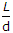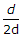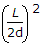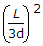# Civil Engineering - Theory of Structures - Discussion

Discussion Forum : Theory of Structures - Section 2 (Q.No. 25)
25.
A simply supported uniform rectangular bar breadth b, depth d and length L, carries an isolated load W at its mid-span. The same bar experiences an extension e under same tensile load. The ratio of the maximum deflection to the elongation, isExplanation:
No answer description is available. Let's discuss.
Discussion:
11 comments Page 1 of 2.

Ajay Kumar Yadav said:   7 months ago
Max Deflection = wl3/48EI.
Elongation = pl/AE.

Now, max deflection/elongation.
=(Wl3/48EI)/(pl/AE).
=(l/2d)2.

B.Singh said:   1 year ago
Perfect explanation, Thank you @Steve.

Anu said:   3 years ago
Perfect explanation, Thanks you @Steve.

Steve said:   5 years ago
The maximum deflection for a simply supported beam is WL^3/48EI.

Strain, e =stress/E

Stress=F/A.
e= F/AE,
but F=WL; e= WL/AE.

The ratio = Max. deflection/Elongation=(WL^3/48EI)/(WL/AE).
But A=b.d; I=bd^3/12.

Therefore we have our ratio as= (WL^3/48E*(b * d^3/12))/(WL/b * d * E)= L^2/4d^2= (L/2d)^2.
(2)

Asraful Adalder said:   5 years ago
Elongation= WL/AE.
Deflection= WL3/48EI.

(WL/b * d * E)/( WL^3/48E * b * d3/12) = (L/2d)2.

Tina said:   5 years ago
Yes, Correct @Himanshu.

Himanshu said:   6 years ago
WL3/48EI = deflection (I= BD3/12).
WL/AE = elongation (A=BD).

Sumit said:   6 years ago
Elongaton is wl/2ae.

Amit said:   7 years ago
Deflection = WL3/48EI (correction).

Vipin said:   7 years ago
Elongation= WL/AE.
Deflection= WL3/EI.

(WL/b * d * E)/( WL3/E * b * d3/12) = (L/2d)2.